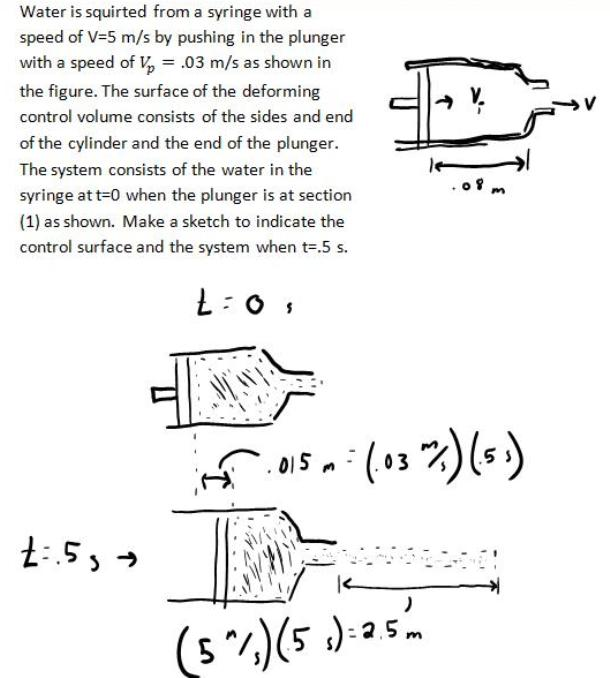fluid mechanics math problems engineering equations fluid mechanics basic mechanics school homework engineering math fluids formulas fluid mechanics problems fluid mechanics problem solutions to fluids problems full solution fluid mechanics engineering fluids problem solution fluid mechanics math problems engineering equations fluid mechanics basic mechanics school homework engineering math fluid mechanics formulas fluid problems fluid mechanics problem solutions to fluid mechanics problems full solution fluid mechanics engineering fluid mechanics problem solution
fluid mechanics math problems engineering equations fluid mechanics basic mechanics school homework engineering math fluids formulas fluid mechanics problems fluid mechanics problem solutions to fluids problems full solution fluid mechanics engineering fluids problem solution fluid mechanics math problems engineering equations fluid mechanics basic mechanics school homework engineering math fluid mechanics formulas fluid problems fluid mechanics problem solutions to fluid mechanics problems full solution fluid mechanics engineering fluid mechanics problem solution
Highalphabet Home Page fluid mechanics solutions fluids math solved Fluid Mechanics Page 1
Water is squirted from a syringe with a speed of V=5 m/s by pushing in the plunger with a speed of V(p)=.03 m/s as shown in the figure. The surafce of the deforming control volume consists of the sides and end of the cylinder and the end of the plunger. The system consists of the water in the syringe at t=0 when the plunger is at section (1) as shown. Make a sketch to indicate the control surface and the system when t=.5 s.Water is squirted from a syringe with a speed of V=5 m/s by pushing in the plunger with a speed of V(p)=.03 m/s as shown in the figure. The surafce of the deforming control volume consists of the sides and end of the cylinder and the end of the plunger. The system consists of the water in the syringe at t=0 when the plunger is at section (1) as shown. Make a sketch to indicate the control surface and the system when t=.5 s.